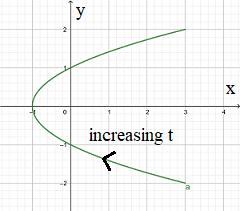# Sketch the curve with the given vector equation. Indicate with an arrow the direction in which t...

## Question:

Sketch the curve with the given vector equation. Indicate with an arrow the direction in which {eq}t {/eq} increases.

{eq}\displaystyle\; \mathbf{r}(t) = \left\langle t^{2} - 1, \;t \right\rangle {/eq}

## Sketch of Parametric Equation:

Let us consider a parametric curve in the plane described by the vector function

{eq}\mathbf r(t) = x(t) \mathbf i + y(t) \mathbf j. {/eq}

A sketch of the curve can be produced by plotting the sequence of points (x,y) when the parameter t varies.

Consider the parametric curve described by the vector function

{eq}\displaystyle\; \mathbf{r}(t) = \left\langle t^{2} - 1, \;t \right\rangle {/eq}

A sketch the curve when the parameter t varies in the range {eq}t \in [-2,2] {/eq} is reported in the figure below

together with an arrow indicating the direction where t increases.